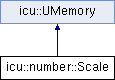ICU 71.1  71.1
icu::number::Scale Class Reference

A class that defines a quantity by which a number should be multiplied when formatting. More...

`#include <numberformatter.h>`

Inheritance diagram for icu::number::Scale:## Public Member Functions

Scale (const Scale &other)

Scaleoperator= (const Scale &other)

Scale (Scale &&src) U_NOEXCEPT

Scaleoperator= (Scale &&src) U_NOEXCEPT

~Scale ()

## Static Public Member Functions

static Scale none ()
Do not change the value of numbers when formatting or parsing. More...

static Scale powerOfTen (int32_t power)
Multiply numbers by a power of ten before formatting. More...

static Scale byDecimal (StringPiece multiplicand)
Multiply numbers by an arbitrary value before formatting. More...

static Scale byDouble (double multiplicand)
Multiply numbers by an arbitrary value before formatting. More...

static Scale byDoubleAndPowerOfTen (double multiplicand, int32_t power)
Multiply a number by both a power of ten and by an arbitrary double value. More...

## Friends

struct impl::MacroProps

struct impl::MicroProps

class impl::NumberFormatterImpl

class impl::MultiplierFormatHandler

class impl::GeneratorHelpers

class ::icu::numparse::impl::NumberParserImpl

class ::icu::numparse::impl::MultiplierParseHandler

## Detailed Description

A class that defines a quantity by which a number should be multiplied when formatting.

To create a Scale, use one of the factory methods.

Stable:
ICU 62

Definition at line 1083 of file numberformatter.h.

## ◆ Scale() [1/3]

 icu::number::Scale::Scale ( const Scale & other )
Stable:
ICU 62

## ◆ Scale() [2/3]

 icu::number::Scale::Scale ( Scale && src )
Stable:
ICU 62

## ◆ ~Scale()

 icu::number::Scale::~Scale ( )
Stable:
ICU 62

## ◆ Scale() [3/3]

 icu::number::Scale::Scale ( int32_t magnitude, impl::DecNum * arbitraryToAdopt )
Internal:
Do not use.

This API is for internal use only.

## ◆ byDecimal()

 static Scale icu::number::Scale::byDecimal ( StringPiece multiplicand )
static

Multiply numbers by an arbitrary value before formatting.

Useful for unit conversions.

This method takes a string in a decimal number format with syntax as defined in the Decimal Arithmetic Specification, available at http://speleotrove.com/decimal

Also see the version of this method that takes a double.

Returns
A Scale for passing to the setter in NumberFormatter.
Stable:
ICU 62

## ◆ byDouble()

 static Scale icu::number::Scale::byDouble ( double multiplicand )
static

Multiply numbers by an arbitrary value before formatting.

Useful for unit conversions.

This method takes a double; also see the version of this method that takes an exact decimal.

Returns
A Scale for passing to the setter in NumberFormatter.
Stable:
ICU 62

## ◆ byDoubleAndPowerOfTen()

 static Scale icu::number::Scale::byDoubleAndPowerOfTen ( double multiplicand, int32_t power )
static

Multiply a number by both a power of ten and by an arbitrary double value.

Returns
A Scale for passing to the setter in NumberFormatter.
Stable:
ICU 62

## ◆ none()

 static Scale icu::number::Scale::none ( )
static

Do not change the value of numbers when formatting or parsing.

Returns
A Scale to prevent any multiplication.
Stable:
ICU 62

## ◆ operator=() [1/2]

 Scale& icu::number::Scale::operator= ( const Scale & other )
Stable:
ICU 62

## ◆ operator=() [2/2]

 Scale& icu::number::Scale::operator= ( Scale && src )
Stable:
ICU 62

## ◆ powerOfTen()

 static Scale icu::number::Scale::powerOfTen ( int32_t power )
static

Multiply numbers by a power of ten before formatting.

Useful for combining with a percent unit:

```NumberFormatter::with().unit(NoUnit::percent()).multiplier(Scale::powerOfTen(2))
```
Returns
A Scale for passing to the setter in NumberFormatter.
Stable:
ICU 62

The documentation for this class was generated from the following file: